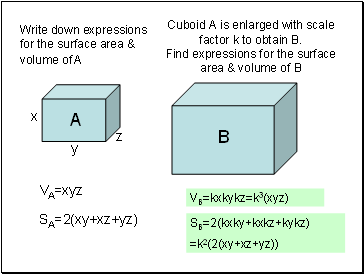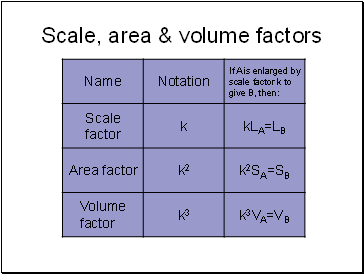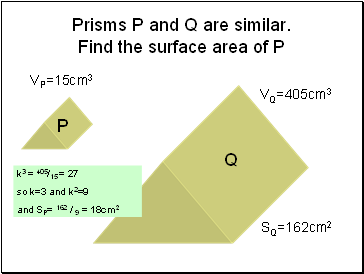# Similar shapesPage 1

#### WATCH ALL SLIDES

Slide 1## Similar shapes

Slide 2Cuboid A is enlarged with scale factor k to obtain B. Find expressions for the surface area & volume of B

y

x

z

A

B

VA=xyz

SA=2(xy+xz+yz)

Write down expressions for the surface area & volume of A

VB=kxkykz=k3(xyz)

SB=2(kxky+kxkz+kykz)

=k2(2(xy+xz+yz))

Slide 3## Scale, area & volume factors

Slide 4Sphere M is enlarged with scale factor 5 to obtain N. Find the volume of N

M

N

VM=20cm3

VN=53x20=2500cm3

Slide 5Cylinders G and H are similar. Find the radius of H

SG=14πcm2

SG=224πcm2

G

H

rG

rG=1.5cm

k2 = 224π/14π = 16

so k=4

and rH= 4x1.5 = 6cm

Slide 6Prisms P and Q are similar. Find the surface area of P

VP=15cm3

VQ=405cm3

SQ=162cm2

P

Q

k3 = 405/15 = 27

so k=3 and k2=9

and SP= 162 / 9 = 18cm2